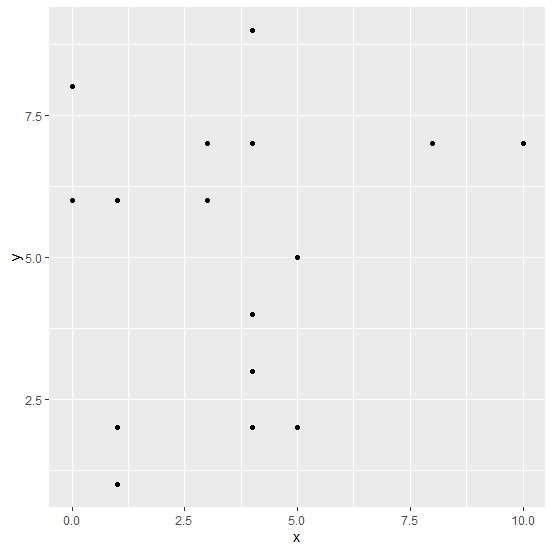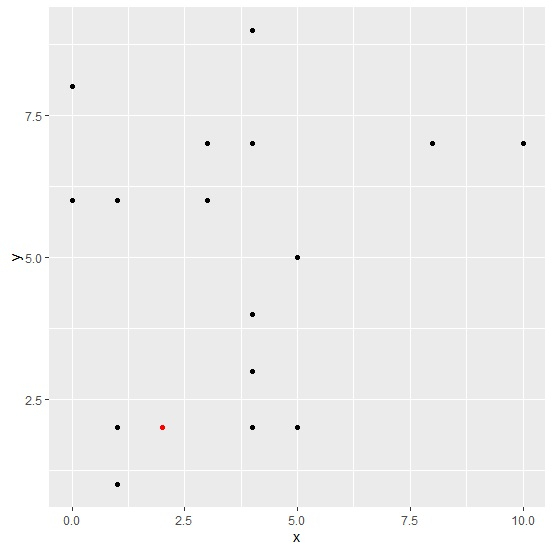# How to add an extra point to scatterplot using ggplot2 in R?

To add an extra point to scatterplot using ggplot2, we can still use geom_point function. We just need to use aes function for quoting with new values for the variables, also we can change the color of this point using colour argument. The display of an extra point will help us to distinguish between a threshold/new value and remaining values.

Consider the below data frame −

## Example

Live Demo

x<-rpois(20,5)
y<-rpois(20,5)
df<-data.frame(x,y)
df

## Output

   x  y
1  4  7
2  3  7
3  4  4
4  8  7
5  4  2
6  0  6
7  5  2
8  3  6
9  0  8
10 4  9
11 4  3
12 10 7
13 4  3
14 1  6
15 1  1
16 5  5
17 1  2
18 4  7
19 4  3
20 4  3

Loading ggplot2 package and creating a scatterplot between x and y −

## Example

library(ggplot2)
ggplot(df,aes(x,y))+geom_point()

## OutputAdding a new point to the above plot −

## Example

ggplot(df,aes(x,y))+geom_point()+geom_point(aes(x=2,y=2),colour="red")

## Output